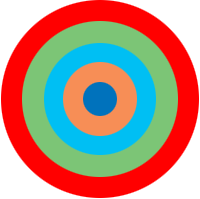SEARCH HOMEMath Central Quandaries & QueriesQuestion from Math, a parent: The bull’s eye of a target is a circle 20 cm in diameter. It is surrounded by four rings, each 15 cm wide. Find the area of each ring.Hi,

I can help get you started. Here is my diagram of the bull's eye, not to scale.The outside circle has a radius of $10 + 15 + 15 + 15 + 15 = 70$ cm. What is the area of a circle of radius 70 cm?

The next smaller circle has a radius of $10 + 15 + 15 + 15 = 55$ cm. What is the area of a circle of radius 55 cm?

The area of the largest ring, the red ring, is the difference between the two areas you just calculated.

PennyMath Central is supported by the University of Regina and The Pacific Institute for the Mathematical Sciences.